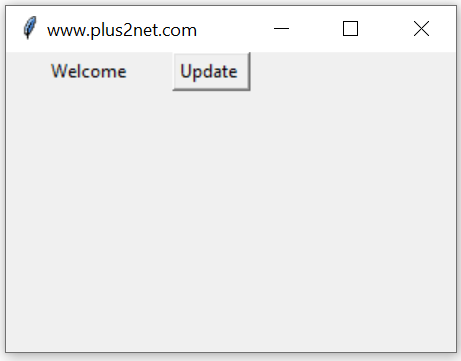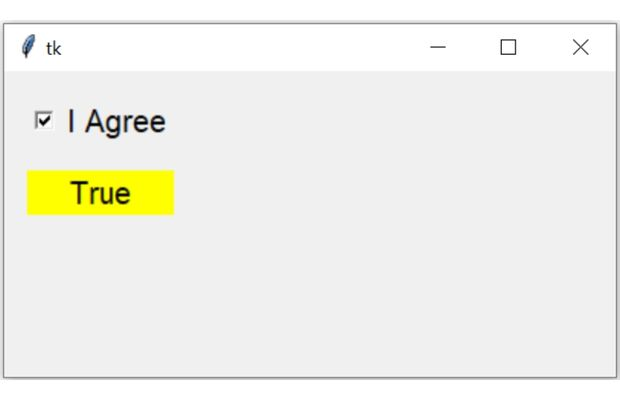# Python tkinter BooleanVar() trace

``c_v=tk.BooleanVar(master,value,name)``
`master`: (Optional)The variable is associated with, default value is parent window.
`value`:(Optional) We can set the initial value for the variable.
`name` : (Optional) Name given default is PY_VAR1

Tkitner BooleanVar() get(), set(), trace() methods to manage data and trigger call back functions

For an BooleanVar() so we can check the different modes of this variable, like this

w :Write- the variable is written by someone.
u :undefined – The variable is deletedHere is an example which uses w ( write ) mode to display the value of the variable when ever it changes.
We used one Button and used on Click event to change the value of this variable bv1 from False to True.
``b1 = tk.Button(my_w,text='Update',command=lambda:bv1.set(True))``
This triggers the trace() which used the callback function my_r() to print the value of the variable ( True ) to our console.
``````import tkinter as tk
from tkinter import *
my_w = tk.Tk()
my_w.geometry("300x100")  # Size of the window

def my_r(*args):
print(bv1.get()) #  Print when variable changes.

bv1 = tk.BooleanVar(my_w) # declare Boolean Variable
bv1.set(0)

b1 = tk.Button(my_w,text='Update',command=lambda:bv1.set(1))

bv1.trace('w',my_r) # monitor the change of variable
my_w.mainloop()``````
Output
``True``
Use StringVar() for handling String data
Use DoubleVar() for handling Float Data
Use IntVar() for handling Integer Data

## set() and get() methods of BooleanVar

In above examples we used set() method to assign data to the Boolean variable and to read the data stored we used get() method. These two methods are frequently used in our scripts.
``````bv1.set(1) # assign value to bv1
print(bv1.get()) # display the value assigned to bv1``````

## Initializing BooleanVar

We can using set() method to assign data to BooleanVar, after declaring or we can assign value while declaring the string variable.
``bv1=tk.BooleanVar(value=True) # Assign value to bv1``

## Length of BooleanVar

Before using len function, we have to convert the BooleanVar to string by using str()
``print(len(str(bv1.get()))) # 4 ``

## Normal Variable and BooleanVar

BooleanVar() is a class in Tkinter. In a GUI application we require various events to be captured to trigger different functions (requirements). If we use normal Python variable with widgets then monitoring the changes is difficult. However by using a BooleanVar we can monitor the changes and trigger functions based on the requirements.

## Reading Checkbuton status using BooleanVarWe will connect the variable option of a Checkbutton to BooleanVar() c_v and read the status.
By using trace() method of Boolean variable we will trigger a call back function.
Inside the function we will read the value of Boolean variable by using get() method and then update the text attribute of a Label by using config() method.
``````import tkinter as tk
my_w = tk.Tk()
my_w.geometry("400x200")  # Size of the window

c_v=tk.BooleanVar()# Declaring the Boolean  variable
c_v.set(1) # Set the value to 2

c1=tk.Checkbutton(my_w,text='I Agree',variable=c_v,
onvalue=1,offvalue=0,font=20)

l1=tk.Label(my_w,text='Output',font=22,bg='yellow')

def my_upd(*args):
my_data=c_v.get() # read the value or state of the Checkbutton
l1.config(text=str(my_data)) # Update the Label with data

c_v.trace('w',my_upd) # On change of Boolean variable trigger function

my_upd() # Update function

my_w.mainloop()  # Keep the window open``````

Subscribe to our YouTube Channel here

## Subscribe

* indicates required
Subscribe to plus2netplus2net.com